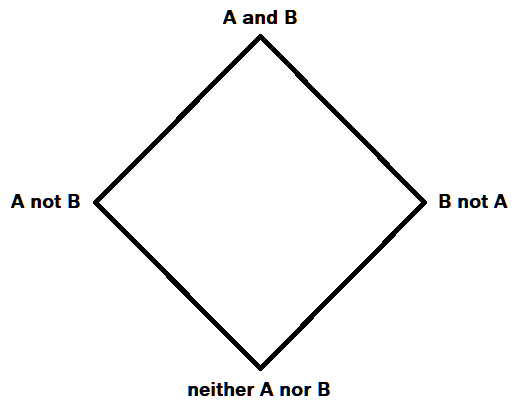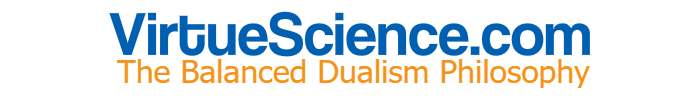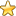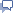You are here: IndexThe Database of Number Correlations# Number Laws

This is a list of laws that apply to the infinite set of whole numbers. I believe that there is a unified theory of mathematics of astounding elegance and beauty. My idea is that as many laws as possible should be listed, then they should be categorised and ordered. Read this article for more information regarding progressive ordering techniques: The Concept Age

So this page is merely the first stage. A lot more laws will need to be added here so if you know of a law or mathematical fact that you think should be listed here than please email me.

 Any multiple of a perfect number or an abundant number is also abundant. Every number greater than 20161 can be expressed as a sum of two abundant numbers. The nth cubic number is a sum of n consecutive odd numbers, for example 1cubed=1, 2cubed=3+5, 3cubed=7+9+11 and 4cubed=13+15+17+19. The sum of the first n odd numbers is a Square Number, for example 1=1, 4=1+3, 9=1+3+5 and 16=1+3+5+7. Any positive integer can be represented in exactly one way as a product of primes. Every positive integer is a sum of at most three triangular numbers. Every positive integer can be written as the sum of at most four squares. The total number of 1s that occur among all unordered partitions of a positive integer is equal to the sum of the numbers of distinct members of those partitions. Reichert and Toepkin (1940) proved that a rectangle cannot be dissected into fewer than nine different squares. It is impossible to fill a rectangular box with a finite number of unequal cubes. No odd Fibonacci number is divisible by 17 All integers are the sum of at most 27 prime numbers. Every positive whole number can be written as the sum of eight cubes (including 0^3 when necessary) EXCEPT 23 and 239. Those two numbers require 9 cubes. Every number is the sum of at most 7 octahedral numbers. There are exactly nine Heegner numbers: 1, 2, 3, 7, 11, 19, 43, 67, 163. Except for 2 and 3, every prime number will eventually become divisible by 6 if you either add or subtract 1 from the number. For example, the number 17, plus 1, is divisible by 6. The number 19, minus 1, is also divisible by 6.

You are here: IndexThe Database of Number CorrelationsShare any properties and meanings for particular Numbers...contact me directly, thanks.Character Improvement The Number Database The Physical Body World Events
The Esoteric Section Tactics and Self Defence Healing Society Conceptual Science
Scientific Theories Webmaster Tips and Tricks Financial Freedom Art, Music, Poetry
Living Space/Environmental Mysteries of the World Non-Duality & Spirituality Shamanism/Magick
Hi, I am James Barton the founder of VirtueScience and Author of "Inner Medicine" which details my discoveries regarding the virtues along with practical exercises to awaken natural virtue. I have a wide range of interests but the main focus of this site now is the virtues and character. Please feel free to explore the site and comment on any pages that you are interested in/agree with or disagree with.